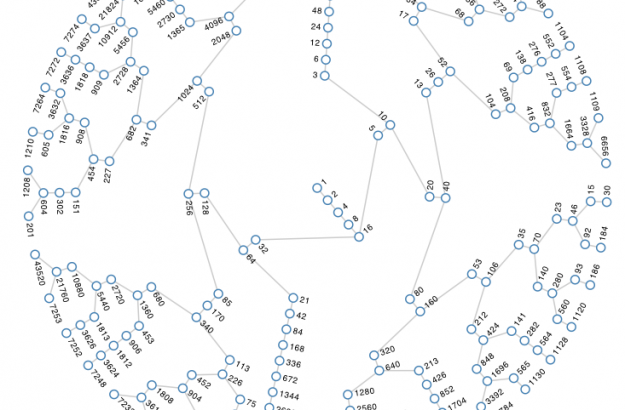## Posts Tagged ‘conjecture’

### All numbers lead to one

04 OctIn 1937, mathematician Lothar Collatz proposed that given the following algorithm, you will always end at the number 1:

1. Take any natural number, n.
2. If n is even, divide it by 2.
3. Otherwise, n is odd. Multiply it by 3 and add 1.
4. Repeat indefinitely.

Developer Jason Davies puts it into reverse and shows all the numbers that fall within an orbit length of 18 or less. Press play, and watch the graph grow. Mostly a fun animation for nerds like me.### All numbers lead to one

04 OctIn 1937, mathematician Lothar Collatz proposed that given the following algorithm, you will always end at the number 1:

1. Take any natural number, n.
2. If n is even, divide it by 2.
3. Otherwise, n is odd. Multiply it by 3 and add 1.
4. Repeat indefinitely.

Developer Jason Davies puts it into reverse and shows all the numbers that fall within an orbit length of 18 or less. Press play, and watch the graph grow. Mostly a fun animation for nerds like me.